You have been redirected to your local version of the requested page

Many different configurations are made possible when using two-, three-, or four-electrode cell setups in research. Depending on the experimental requirements, one setup may be preferred over another. Therefore, the proper electrode arrangements for these three situations are defined in this Application Note.

As an example, the potential at the counter electrode is measured during the platinum oxidation in acidic media, with the second sense (S2) of VIONIC powered by INTELLO. Since dissolved Pt in solution could bias the results, it is important to be able to monitor the potential of the counter electrode.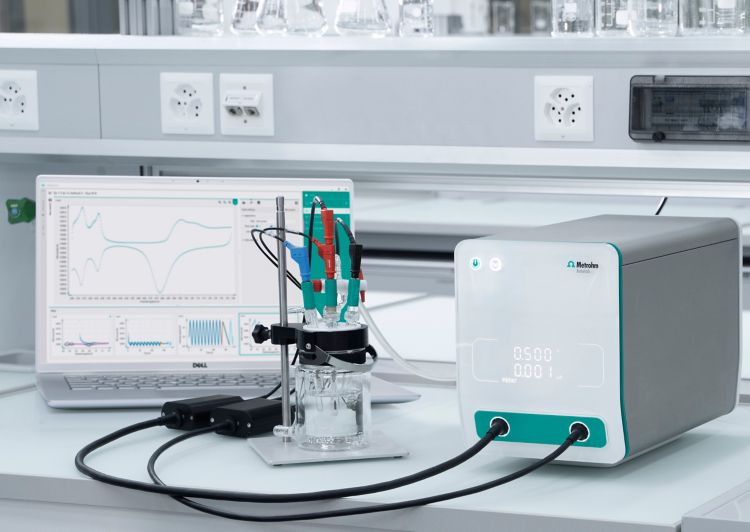When using a PGSTAT set in potentiostatic mode, the desired potential is applied between counter and working electrodes. At the working electrode, the current is also measured. The potential is measured as difference between the potential at the sense (S) and reference (RE) leads, 𝑉S − 𝑉RE.

For some experiments, measuring only one potential in an electrochemical cell is not sufficient enough to form a complete picture of the occurring phenomena.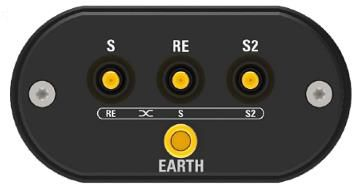Figure 2. Shown here is the buffer box (part of the Pure Signal Bridge) with connections for the EARTH ground, as well as the Adaptive Cables of the sense (S), reference (RE), and second sense (S2).

The buffer box of the Pure Signal Bridge contains the connections for the EARTH ground and for the Adaptive Cables of the sense (S), reference (RE), and second sense (S2), Figure 2.

If the potential is measured with S2, the value is calculated as the difference of the potential measured at the reference and S2, 𝑉S2 − 𝑉RE.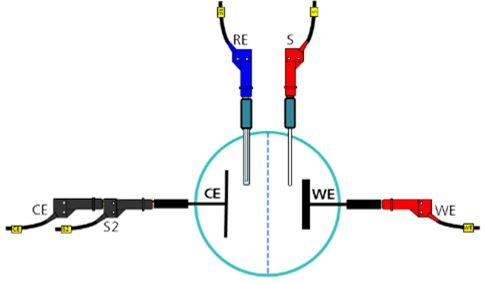Figure 3. Electrode arrangement in the case of using a four-electrode cell.

In a four-electrode cell, the reference lead is connected to a reference electrode and the sense lead is usually connected to a platinum electrode. The S2 is usually connected to the counter electrode (Figure 3).

In this way, the potential difference between the reference and sense electrodes is measured and controlled, while the potential between reference and counter electrodes is only measured.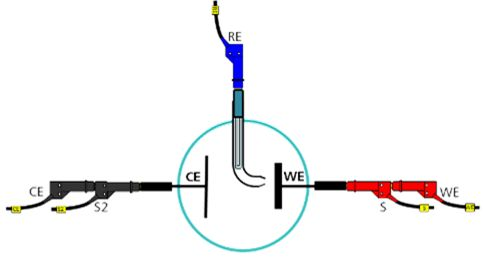Figure 4. Electrode arrangement in the case of using a three-electrode cell.

In a three-electrode cell configuration, the reference lead is connected to the RE, while the sense lead is connected to the working electrode. The S2 is connected to the counter electrode (Figure 4).

With this setup, the potential difference between the reference and sense electrodes is measured and controlled, while the potential between reference and counter electrodes is only measured.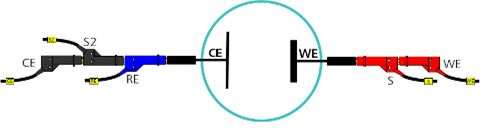Figure 5. Electrode arrangement in the case of using a two-electrode cell.

When using a two-electrode cell, the reference electrode lead is connected to the counter electrode (CE), while the sense lead is connected to the working electrode (Figure 5).

Here, the potential difference between the two electrodes is measured between the RE and S leads. As shown in Figure 5, it is possible to connect the S2 lead to the counter electrode, together with the reference lead. However, the PGSTAT will measure a potential of 0 V, as the potential difference between two leads connected together is 0 V.

Electrocatalysis is just one of the applications where measuring a second potential is necessary. The potential of a platinum counter electrode 𝑉𝐶𝐸 is measured together with the potential of a platinum working electrode 𝑉𝑊𝐸 during cycling voltammetry (CV) of a Pt working electrode in acidic media.

Here, it may occur that the potential at the counter electrode is high enough to allow the electrodissolution of platinum. Dissolved platinum in solution could interfere with the process occurring at the WE and bias the results. It is thus important to be able to monitor the potential of the counter electrode.

In this Application Note, the potential at the counter electrode is measured together with the potential at the working electrode, and the results are compared.

The experiments were carried out with a Metrohm Autolab VIONIC powered by INTELLO. The VIONIC instrument is equipped with a linear scan generator as standard, allowing the possibility to perform linear sweeps and linear cyclic voltammetries (CVs).

A Metrohm Pt wire was used for the working electrode, and a Metrohm Pt sheet electrode was used for the counter electrode. A Metrohm Ag/AgCl 3mol/L KCl was used for the reference electrode. An Autolab Electrocatalysis RRDE cell was employed and placed inside of a Metrohm Faraday cage.

The INTELLO procedure began by polarizing the working electrode to 0.15 V. After 3 seconds of stabilization time, a linear potentiostatic CV followed. The CV consisted of three scans, starting at 0.15 V, scanning up to the first vertex at 1.35 V, then scanning down to the second vertex at -0.81 V, and finally ending again at 0.15 V. The scan rate was 500 mV/s, and the potential interval was 5 mV, creating a sampling interval of 10 ms.

In Figure 6, the resulting cyclic voltammograms are shown. The data points are shown in a gradient of blue, depending on the scan. Lighter colored data points are from the first scan, medium colored points are from the second scan, and the dark colored points are from the third scan.

Figure 6. The cyclic voltammogram of the linear CV on the Pt electrode in sulfuric acid. The first scan is in light blue, the second scan is in blue, and the third scan is in dark blue.

A discussion of the cyclic voltammogram can be found in the Metrohm Application Note AN-EC-025.

Application Note AN-EC-025

In Figure 7, the time plot of the WE potential in orange and the CE potential in blue is shown. Light colors (left) correspond to the first scan, intermediate colors are from the second scan, and dark colors (right) are from the third scan.

Figure 7. Time plot of the WE potential (orange) and the CE potential (blue). Light color gradients (left) are from the first scan, intermediate color gradients are from the second scan, and dark color gradients (right) come from the third scan.

Figure 7 shows that the potential at the counter electrode (blue) reaches values higher than 0.6 V, but this is not sufficient enough to facilitate a substantial Pt dissolution.

In this Application Note, the capabilities of VIONIC powered by INTELLO in measuring the potential at the counter electrode together with the potential at the working electrode, were demonstrated by studying platinum oxidation in acidic media.

1. L. Xing et al., Electrocatalysis (2014)5:96–112